# How Big Should the Control Group Be in a Randomized Field Experiment?

Research involves trade-offs. Basic social science—aimed at scientific discovery and theory-building—is dealing with a “replication crisis,” and much of the debate between scholars stems from people valuing costs and benefits differently. Some believe false positives are more dangerous to scientific advancement than are false negatives, while others feel the opposite. This debate centers around trade-offs: If we have a stricter threshold for determining what is scientific evidence, we are going to make fewer wrong proclamations that an effect or relationship exists—but we are also going to miss out on interesting scientific discoveries. If we impose a looser threshold, the opposite is true.

Social science in the real world involves additional, practical trade-offs that researchers and data scientists must manage. Such is the case when considering the current question of how large a control group should be in a randomized field experiment. For the purposes of this post, I consider an experimental design where participants are assigned to one of two conditions: a treatment or a control. I am defining the size of a control condition relative to the size of the sample: the proportion allocated to the control condition.

Tensions in this situation involve the same as those in basic research—in addition to others. Randomized field experiments meet people where they are, in their day-to-day lives. This often requires considerable more resources than forcing a college sophomore to show up to your lab between classes. And the stakeholders invested in whatever it is we are testing—be it a technology, campaign, technique, or strategy—do not want to miss an opportunity we have to engage with people.

This is especially true in politics. Consider a field experiment testing the effect an advertising or canvassing campaign has on voter turnout. Every person we allocate to the control condition is a potential voter with whom we do not speak. People who work hard to design advertisements want people to see their work; volunteers want to knock on doors and talk to people about the candidate they support. Elections only happen once, as well; we cannot go back later and contact the people who were in the control condition.

These are just some of the trade-offs researchers and data scientists must consider in conducting field experiments. We want valid statistical inferences. We want to efficiently quantify the causal effect of these campaigns. It is clear to us that half of the participants should be in the treatment condition, while the other half is in the control condition, as this strategy gets us equally-precise estimates of the mean or frequency of the dependent variable in both conditions. But this means that we do not engage with a full 50% of the people we are targeting. So how many should we target? How can we manage these practical and methodological considerations? At the other extreme, we could expose 99.5% of the sample to the treatment and only 0.5% to the control. We are engaging the vast majority of people. And while we would have a very precise estimate of what is happening in the treatment condition, the standard error in the control condition would be so large that we would be clueless as to if it meaningfully differs from the treatment.

The figure below illustrates this. I simulated data from an N = 100 experiment where 50 people were in each condition (“50/50”) or only 5 were in the control (“95/5”). Even though the effect is bigger in the latter situation, we cannot detect a significant effect—there are not enough people in the control condition (p = .102). However, there is a significant effect found with the 50/50 split (p = .015).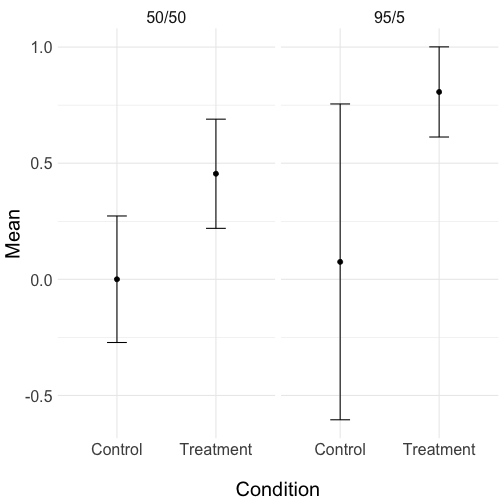We could employ a mental calculus in determining the size of the control condition, weighing the opportunity costs associated with not exposing someone to the treatment against the statistical efficiency of an even split between the conditions. This might serve us reasonably well, but my goal here is to quantitatively inform this calculus through a Monte Carlo simulation study, examining the relationship between statistical power and control group size. I now turn to discussing the methods of this study (i.e., how the simulation was done) before discussing results and implications.

## Method

I decided each simulated dataset would mimic a randomized field experiment on voter turnout. Cases were assigned to either a “treatment” or a “control” condition. The outcome was either 1 (“Voted”) or 0 (“Did Not Vote”). As these are simulated data, this could represent any other dichotomous outcome, such as 1 (“Favorable”) or 0 (“Unfavorable”), etc.

Second, I defined a range of characteristics for the data. These were:

1. Sample size: N = 1000, 2500, 5000, 10000, 15000, or 20000.

2. Control group size: 10%, 15%, 20%, 25%, and so on, up to 50% of the sample.

3. Effect size: This was defined by lift, which is the percentage point increase in the positive outcome (e.g., voting, favorable opinion, donating, etc.) that the treatment had over the control. If 70% of people voted in the control condition and 72% did so in the treatment, this would represent a lift of 2 percentage points. Note that power depends on the rate of the positive outcome in the control condition. I set this constant across all datasets at 50%. This represents the best case scenario for power, given that power decreases as this control date approaches 0% or 100%. In preliminary simulations, however, I allowed this base rate to be 30%, 50%, or 70%. The this did not change the shape of the relationship between control size and power; it merely shifted the intercept up or down a little bit. Data from those preliminary simulations can be found at GitHub.

Third, I simulated 1,000 datasets for each of the 540 combinations of the characteristics above (i.e., 6 sample sizes times 9 control sizes times 10 effect sizes). For each dataset, cases were assigned to treatment or control conditions deterministically based on the control group size. If we let the size of the control group, Ctl, be the proportion of the sample in the control condition, and the size of the sample to be N, then N x Ctl cases were in the control, while N x (1 - Ctl) were in the treatment condition. For the control condition cases, the dependent variable for each case was drawn from a Binomial distribution, B(1, 0.5), while the treatment case outcome variables were drawn from a distribution B(1, 0.5 + L), where L denotes the lift.

Fourth, p-values were obtained by regressing the outcome on the condition in a binomial logistic regression. For each of the 540 types of datasets, I calculated the proportion of the 1,000 simulations that yielded a p-value below .05. This represents the statistical power for that combination of data characteristics: It estimates the percentage of the time we would find a significant effect, given that it exists at that effect size. For example, if 800 of the 1,000 simulations yielded p < .05, then the power for that combination of data characteristics would be 80%.

The resulting data I analyzed to examine the relationship between control size and power was one with 4 variables (N, control size, lift, and power) and 540 cases (each unique combinations of the N, control size, and lift possibilities).

## Results

I started by generating curves illustrating the relationship between control size and power for each of the 60 N x Lift combinations, which are found below. All curves in the following analyses, unless where otherwise noted, were fitted using a natural cubic spline with 3 degrees of freedom (via the `mgcv` and `splines` R packages); all graphing was done using `ggplot2`.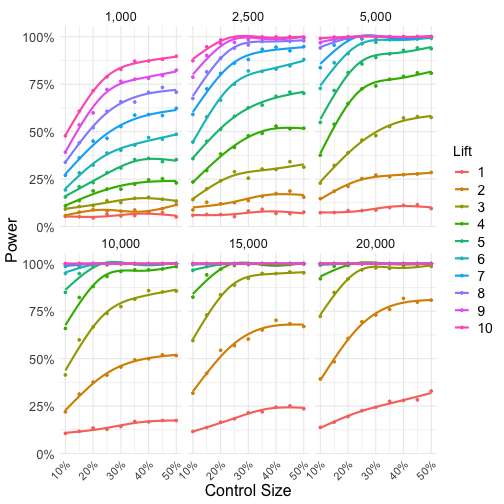The first lesson from these curves is that deviating from a 50/50 treatment and control split harms statistical power. This is true in just about every circumstance, except when analyses are so tremendously over or underpowered that it does not matter.

Below the 20% control size mark, even analyses that have about 100% power in the 50/50 case can start to lose power. Consider the 4 point lift curve when N = 20,000 (bottom right). Even though the study has about 100% power when holding out only 20% of the sample as control cases, this starts to tail off below 20% (granted, most researchers would still be happy with the level of power at the 10% control size).

Most of these curves, however, do not represent typical situations data scientists face. The lower bound of power generally seen as “acceptable” is 80%, so we try to achieve at least that. We seldom find ourselves in situations when we have greater than 95% power, either. To get a more realistic picture, I now turn to only looking at the curves that achieve between 80% and 95% power when the control size is half of the sample. The horizontal dotted lines represent these 80% and 95% thresholds.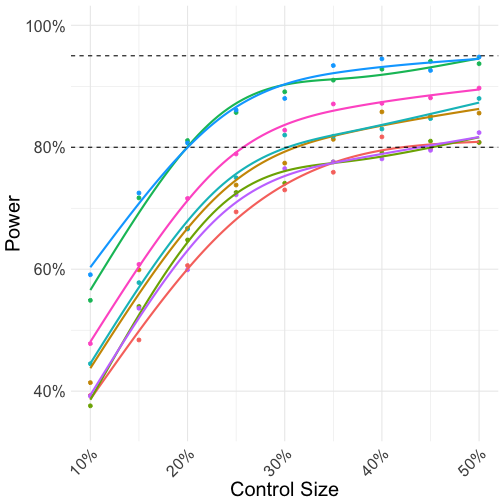If we were already teetering on having 80% power in the 50/50 split situation, we lose that once we dip below about a 40% control size. Since these curves otherwise follow the same form, I decided to just fit one curve to all of these data points, collapsing across the N x Lift combinations.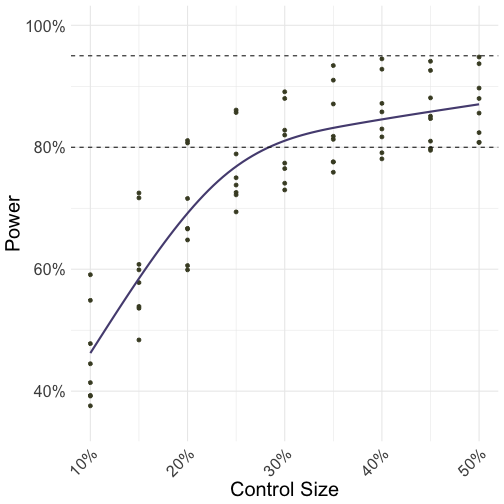Things start to dip once we go below the 30% control size mark, and the 80% power threshold is crossed just below that point. Just by looking at the curve, I guessed that power really starts to drop off around 25% to 30% control size. To be a bit more precise than eyeballing, I fit a piecewise linear regression to these data (via the `segmented` R package). This involves fitting one straight line on cases above a certain threshold and another straight line on cases below it. Doing so allows us to get an idea of a single breakpoint where the relationship between control size and power changes.

But where do we set the breakpoint? Estimation requires the analyst to take a guess at where it might be, and an iterative procedure searches around this until it converges on a solution that fit the data well. I specified my initial guess at a control size of 30%. The breakpoint was estimated at 23.2%, with a 95% confidence interval from 19.5% to 26.9%. I think of this as the “elbow” of the curve, where loss of power accelerates. In reality, there is not truly one turning point—the loss of power follows a smooth curve. But estimating this breakpoint gives us a useful approximation. It helps us have a mental benchmark of an area we should not go below when determining the size of control groups.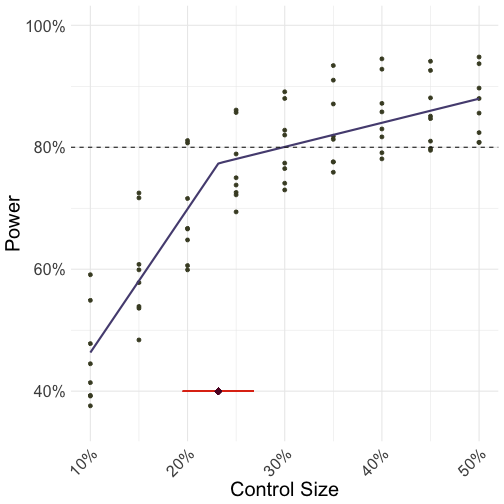## Takeaways

The best scenario, statistically-speaking, is an even split between treatment and control.

We must often consider many factors outside of the research design and statistical world; research involves trade-offs. The decision of how many people to allocate to a control group should be based on a collaboration between relevant parties involved in the research process. In addition to this collective judgment on the opportunity costs of not exposing participants to the treatment, some important takeaways from this simulation study to remember are:

1. Minimal losses in power occur when we shrink the control size to 40%.

2. A 25% to 30% range is a good compromise, as this exposes 70% of the sample to the treatment, yet still does not harm power terribly.

3. You should not allocate less than 20% of the sample to the control condition, save for situations when you are looking for large effects (e.g., 8 point lifts) and/or using large samples (e.g., 15,000 participants).

I did not perform an exhaustive simulation of all possible scenarios, of course. If you would like to examine a case specific to your interests, explore these data, or replicate the results, all of the code can be found at GitHub.

# On Calculating Power for Interactions in 2 x 2 Factorial Designs

Link to the power calculation app.

Note: I have made a few updates to the app since originally publishing this blog post, including making the visuals prettier and including a field to adjust the alpha level. One can track updates at the GitHub repository, https://github.com/markhwhiteii/power_twoway.

## Introduction

Statistical power is important when doing experimental psychology. I'm not going to try to convince you of this—I think there is enough published work over the last few decades that should do so. Instead, I'm going to assume you want your study to be adequately powered and you are trying to figure out how many participants you need to find the interaction you hypothesize. I'm going to show what I think is an intuitive way of conducting a power analysis for an interaction effect in a 2 x 2 between-subjects experiment.

### Background

Westfall notes how people can be confused when they go to G*Power and it tells them they need the same amount of participants in a 2 x 2 design as they do in a simple randomized experiment with two conditions. I'll leave it to the three blog posts linked above to explain this dilemma.

Simonsohn and Giner-Sorolla both offer rules-of-thumb in sample size planning for a 2 x 2 interaction that sound something like: "In Situation X, you should multiply your per-cell sample size from Study 1 by Y," where Study 1 was a between-subjects experiment with two conditions. I agree with Westfall that we should not necessarily be thinking about cell size, but overall N. I also don't like using the a priori sample size from Study 1 to inform the sample size for Study 2, as this ignores the information we learned in Study 1. And using G*Power is difficult, because I don't really know how many people think of interesting, real-world phenomena and say to themselves, "Ah yes, this is probably a .15 Cohens f-squared!"

What I find intuitive is sketching out a pattern of means you expect to see and then calculating power for those results, which is what I suggest one does when calculating power for an interaction effect in a 2 x 2 between-subjects design.

### THE CURRENT APPROACH

I'm going to assume familiarity with Cohen's d—that is, how many standard deviations two means are from one another. If you understand that, you can understand this approach.

Imagine we have a dependent variable that has a standard deviation of 1. That means that any mean differences we find are standardized differences—that is, they are in units of Cohen's d. If one mean is 0.2 and another is 0.7, the Cohen's d between these two means is 0.5. With this in mind, the steps I suggest (and coded into a tool) are:

1. For your 2 x 2 design, sketch out four means you expect to see, assuming that the dependent variable in all conditions has a standard deviation of 1. Use an observed Cohen's d to inform you of this.

2. Get an overall sample size and simulate data based on these means and sample size.

3. See if the p-value for the interaction effect is less than .05.

4. Do steps 2 and 3 a large number of times.

5. Get the proportion of times your simulated data had a p-value less than .05. This proportion is the power for that sample size.

6. Do this for a wide range of possible overall sample sizes.

The logic behind simulating data is that frequentist statistics are all about the long-run: If we were to do this exact study a large number of times, what proportion of the time would we find a significant effect? What the computer can do is simulate this long-run and tell you what proportion that is significant—that is, the power.

I have written a few R functions that accomplish the 6 steps above so that you don't have to (the functions are located here: https://github.com/markhwhiteii/power_twoway/blob/master/helpers.R).

I also wrapped these R functions into a Shiny web app, which you can access here: https://markhw.shinyapps.io/power_twoway/.

Basically, what the backend code does is: first, randomly assign cases to Level 1 or Level 2 of two factors (cleverly named Factor 1 and Factor 2); second, draw values of the dependent variable for these cases from a population with a standard deviation of 1 and a mean of whatever you input for that specific cell; third, run the model and see if we got a significant interaction effect. I do this for a variety of sample sizes that you input and for how many simulations that you specify. The proportion of significant effects found is the estimated power.

I'll walk through the three interaction examples Giner-Sorolla discussed in his post: the reversal, the knockout, and the attenuation. For all of these examples, imagine we conducted a Study 1 that was a simple randomized between-subjects experiment with two conditions and found a Cohen's d of .44. Now, we are interested in throwing another manipulation in there in Study 2 (to make a 2 x 2 design) and looking for an interaction.

## Examples

### The Reversal

This happens when you are expecting the effect found in Study 1 to be reversed in another condition. In the present case, we predict a Cohen's d of .44 in one condition and a Cohen's d of -.44 in another. Let's assume that there are no main effects here. So what we can say is:

• The mean for participants in Factor 1, Level 1 and Factor 2, Level 1 is .22

• The mean for participants in Factor 1, Level 1 and Factor 2, Level 2 is -.22

This means that when Factor 1 is at Level 1, there is a Cohen's d of .44 between Levels 1 and 2 of Factor 2 (because .22 - (-.22) = .44). But now let's add in Factor 1, Level 2:

• The mean for participants in Factor 1, Level 2 and Factor 2, Level 1 is -.22

• The mean for participants in Factor 1, Level 2 and Factor 2, Level 2 is .22

See how the difference between levels in Factor 2 has completely flipped for Level 2 of Factor 1—the effect is d = -.44 ( because -.22 - .22 = -.44). The app will show you what this pattern of means looks like:

We can look at sample sizes from 100 to 400 by 25 (i.e., 100, 125, 150, 175, 200, etc.) and do 300 simulations per sample size. The inputs for the app look like:

Then we can check out the results it returns:

We get 80% power somewhere between 150 and 175 participants. One could now adjust the overall sample size between minimum of 150 and maximum of 175, stepping by 1 each time, to see about how many participants they need.

### The Knockout

Now, imagine that we are expecting a manipulation to completely wipe out the effect we found in Study 1. The means would look like:

• The mean for participants in Factor 1, Level 1 and Factor 2, Level 1 is .00

• The mean for participants in Factor 1, Level 1 and Factor 2, Level 2 is .44

This captures the Cohen's d = .44 we found in Study 1. Then we think Level 2 of Factor 1 is going to bring everyone down to .00:

• The mean for participants in Factor 1, Level 2 and Factor 2, Level 1 is .00

• The mean for participants in Factor 1, Level 2 and Factor 2, Level 2 is .00

The pattern of means, per the app, looks like:

We can then run the power analysis leaving all of the other presets the same. Bad news this time: Even a sample size of 400 only gets us at 60% power!

And this might be too generous of a prediction, as well. The effect of .44 found in Study 1 (or Level 1 of Factor 1 in the plot above) is almost assuredly not coming from one source. If we knock out a hypothesized source of the effect, we should only expect to attenuate the effect, since other sources of the effect still exist. We can look at that next.

### The Attenuation

Let's assume we can only get rid of half of the effect. We would plug in:

• The mean for participants in Factor 1, Level 1 and Factor 2, Level 1 is .00

• The mean for participants in Factor 1, Level 1 and Factor 2, Level 2 is .44

• The mean for participants in Factor 1, Level 2 and Factor 2, Level 1 is .00

• The mean for participants in Factor 1, Level 2 and Factor 2, Level 2 is .22

Which looks like:

Even worse news this time: We are only getting to about 20% power at best in the 350 to 400 range.

## Conclusion

This is, I think, an intuitive way of going about sample size planning for interaction effects in 2 x 2 between-subjects designs. Think about the Cohen's d of the pairwise comparisons you are interested in, come up with means that represent those, and then simulate data based on those means using the app (https://markhw.shinyapps.io/power_twoway/).

## Appendix

A few technical notes:

1. You might find that a larger sample size is giving you lower power. Why? It is likely because you are not using enough simulations to give you a stable power estimate. You can up the number of simulations to do—but know that it will take longer. I suggest starting with a wide range of possible sample sizes and a lower number of simulations (a few hundred), figuring out a promising range, and then going back to just that range with a higher number of simulations.

2. I hard-coded a set.seed() statement in the app, which means that—as long as you enter the exact same inputs—you'll get the same results every time.